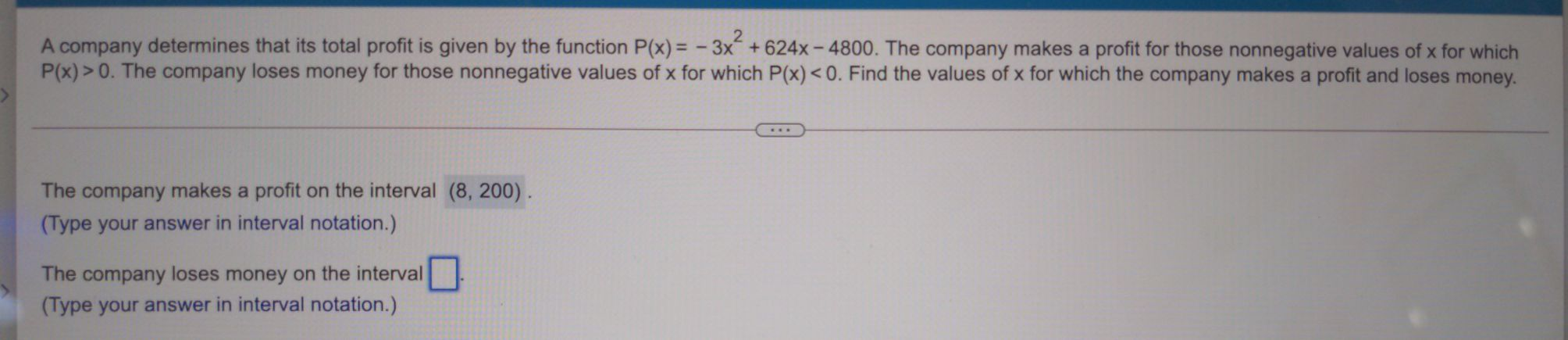### ¿Todavía tienes preguntas de matemáticas?

Pregunte a nuestros tutores expertos
Algebra
PreguntaA company determines that its total profit is given by the function $$P ( x ) = - 3 x ^ { 2 } + 624 x - 4800$$ . The company makes a profit for those nonnegative values of $$x$$ for which

$$P ( x ) > 0$$ . The company loses money for those nonnegative values of $$x$$ for which $$P ( x ) < 0$$ . Find the values of $$x$$ for which the company makes a profit and loses money. The company makes a profit on the interval $$( 8,200 )$$ . (Type your answer in interval notation.) The company loses money on the interval (Type your answer in interval notation.)

$$(- \infty,8 )\cup (200,\infty )$$